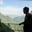Related Tags

r programming
communitycreator

# How to read Excel files in RAKASH BAJWA

### Overview

For simple data analysis, the first important step after data scrapingthe process of importing information from a website into a spreadsheet or local file saved on your computer. is to load data into memory through any programming language for preprocessing.

Therefore, we will discuss two techniques to read an Excel file in R.

### Technique 1: Use read_excel() from readx1

The read_excel() function is used to read an Excel file, and it is only accessible after you import the readxl library in the R program.

### Syntax

read_excel(
path,
sheet = 1,
col_names = TRUE,
col_types = NULL,
na = "",
skip = 0
)


### Parameters

• path: The directory of the Excel file with extension.

• sheet: Default= 1. If we have more than 1 sheet in a single workbook, we can assign sheet #, i.e., 1, 2, 3, 4…

• col_names: Default= TRUE.

1. TRUE: Read the first row as a file header.
2. FALSE: The file does not have a header.
3. Character Vector: Character vector that contains header names.
• col_types: Default= NULL.

1. NULL: Interpret the type of column from the spreadsheet, i.e., Date, Currency, etc.
2. Vector: A vector that contains "blank", "numeric", "date", or "text".
• na: Default= "". Keeps empty cells as is, but we can specify na to fill empty cells.

• skip: Default= 0. Number of rows to skip from start before reading a file.

### Return value

This method returns Excel file data as a data frame.

### Code

#### Example 1

In this example, we read an Excel file, Employee.xlsx. Below, we have the expected output of this code.

main.r
Employee.xlsx
# installing library

print(data)

### Technique 2: Use read.xlsx() from xlsx

The read.xlsx() function is imported from the xlsx library, which is used to read an Excel file in R language.

### Syntax

read.xlsx(
path,
sheet,
startRow = 1,
colNames = TRUE,
rowNames = FALSE,
detectDates = FALSE,
skipEmptyRows = TRUE,
skipEmptyCols = TRUE,
rows = NULL,
cols = NULL,
check.names = FALSE,
sep.names = ".",
namedRegion = NULL,
na.strings = "NA",
fillMergedCells = FALSE
)


### Parameters

• path: Directory of file including extension.

• sheet: Index or name of the sheet to read data.

• startRow*: Default= 1. The first row to begin and check for data.

• colNames: Default= TRUE. If True, the first row will be selected as column names.

• rowNames: Default= FALSE. If True, the first column will be selected as row names.

• detectDates: Default= FALSE. If True, this method will try to recognize Dates and perform conversions if needed.

• skipEmptyRows: Default= TRUE. If True, empty rows will be skipped during loading. After the first row containing data, other empty rows will be returned as NAs.

• skipEmptyCols: Default= TRUE. If True, empty columns will be skipped during loading.

• rows: Default= NULL.

• NULL: If rows are set to NULL, then all rows are read.

• Vector: If rows are set to a vector of numeric values, then specified rows will be read.

• cols: Default= NULL.

• NULL: If cols is set to NULL, then all columns are read.

• Vector: If cols is set to vector of numeric values, then specified columns will be read.

• check.names: Default= FALSE. If True, then it will check if each variable is syntactically valid or not.

• sep.names: Default= ".". Separates each variable in column names.

• namedRegion: Default= NULL.

• NULL: Values of rows, cols, and startRow parameters will be considered within a workbook.

• Not NULL: Values of rows, cols, and startRow parameters are ignored.

• na.strings: Default= "NA". Returns blank cells as NAs.

• fillMergedCells: Default= FALSE. If True, then the value in a merged cell (Parent) is given to all cells within the merge (Child).

### Return value

This method returns Excel file data as a data frame.

#### Example 2

main.r
Employee,xlsx
# Installing required library
install.packages("xlsx") # Optional step

print(data)`

RELATED TAGS

r programming
communitycreator

CONTRIBUTORAKASH BAJWA
RELATED COURSES

View all Courses

Keep Exploring

Learn in-demand tech skills in half the time